# Constructors of Thread class in C#

## Constructors of Thread class in C#

In this article, I am going to discuss the Constructors of Thread class in C# with some examples. Please read our previous article before proceeding to this article where we discussed the basics of Multithreading in C# with examples. As part of this article, we are going to discuss the following pointers in detail with examples.

1. Understanding the Constructors of Thread Class in C#.
2. Why the constructor of Thread class taking a parameter of Delegate type?
3. Understanding ThreadStart delegate in C#.
4. Thread Function with Parameter in C#.
5. Understanding ParameterizedThreadStart Delegate in C#.
7. What are the Problems with the ParameterizedThreadStart delegate in C#?
8. How to Overcome the Problems of ParameterizedThreadStart delegate in C#?
##### Understanding the Constructors of Thread Class in C#.

Let us understand this with an example. Please have a look at the following example.

```using System.Threading;
using System;
{
class Program
{
static void Main(string[] args)
{
t1.Start();
}

static void DisplayNumbers()
{
for (int i = 1; i <= 5; i++)
{
Console.WriteLine("Method1 :" + i);
}
}
}
}
```

As you can see in the above example, we created an instance of the Thread class and to the constructor of the Thread class, we passed the method name that we want the thread to execute as shown below.

##### Constructors of Thread Class in C#:

In C#, the Thread class contains four constructors. If you go to the definition of Thread class then you can see the Constructors as shown below.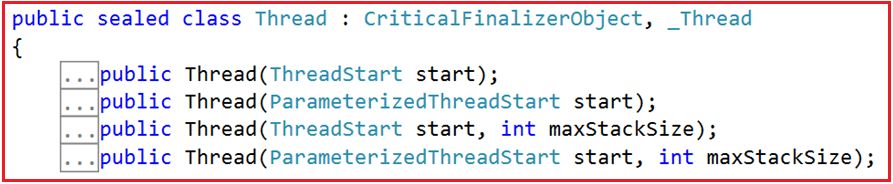Now you may have one question, the Thread class constructor which takes one parameter is either of the type ThreadStart or ParameterizedThreadStart, but in our example, we are passing the method name as a parameter to the Thread class Constructor and it works, how?

To understand this, let’s go to the definition of ThreadStart and you can see ThreadStart is a delegate as shown below.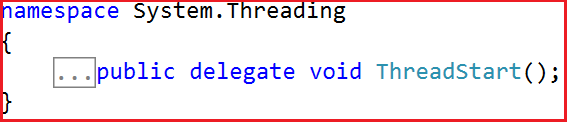##### Why the constructor of Thread class taking a parameter of Delegate type?

As we already discussed, the main objective of creating a Thread in C# is to execute a function. A delegate is a type-safe function pointer. It means the delegate points to a function that the thread has to execute. In simple words, we can say that all the threads that we create require an entry point (i.e. a pointer to the function) from where it should execute. This is the reason why threads always require a delegate. If you want to learn Delegates in C# with examples, then I strongly recommended you to read the following article where we discussed Delegates in detail.

Delegates in C# with Examples

Note: The signature of the delegate should be the same as the signature of the method it points to.

The ThreadStart delegate does not take any parameter as well as the return type is void. In our example, the DisplayNumbers() function signature is the same as the ThreadStart delegate signature as this function return type is void as well as it does not take any parameters.

The above statement implicitly converted to ThreadStart delegate instance. So, you can write the above statement as shown below.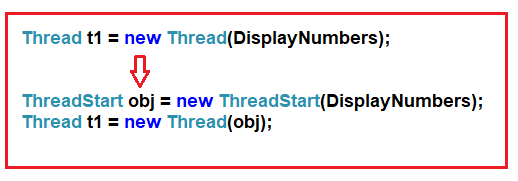It’s a two steps process. First, we need to create the ThreadStart delegate instance and while creating the instance to its constructor we need to pass the method name which we want to execute. In the second step to the Constructor of Thread class, we need to pass the ThreadStart instance as a parameter.

##### The Complete Example is given below:
```using System.Threading;
using System;
{
class Program
{
static void Main(string[] args)
{
//Creating the ThreadStart Delegate instance by passing the
//method name as a parameter to its constructor

//Passing the ThreadStart Delegate instance as a parameter
//its constructor

t1.Start();
}

static void DisplayNumbers()
{
for (int i = 1; i <= 5; i++)
{
Console.WriteLine("Method1 :" + i);
}
}
}
}```

You can also combine the above two statements into a single statement as shown below.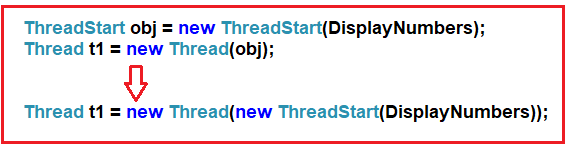It is also possible to create a Thread class instance using the delegate keyword as shown below.

We can also rewrite the same line using an anonymous method as shown below.

You can also directly write the logic as part of the anonymous method instead of calling the DisplayNumbers method as shown below.

```Thread t1 = new Thread(() => {
for (int i = 1; i <= 5; i++)
{
Console.WriteLine("Method1 :" + i);
}
});
```
##### Thread Function with Parameter in C#:

Let change the DisplayNumbers() method implementation. Now, this method takes one input parameter of the object type. And then convert that object type to an integer value and then print the numbers up to that value starting from 1.

```using System.Threading;
using System;
{
class Program
{
static void Main(string[] args)
{
Program obj = new Program();
t1.Start(5);

}

public void DisplayNumbers(object Max)
{
int Number = Convert.ToInt32(Max);
for (int i = 1; i <= Number; i++)
{
Console.WriteLine("Method1 :" + i);
}
}
}
}
```

When the method taking one parameter, then the Thread class uses the ParameterizedThreadStart delegate. The definition of ParameterizedThreadStart is shown below.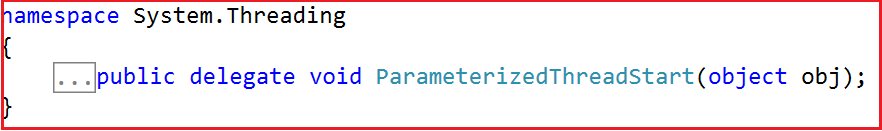As you can see the ParameterizedThreadStart delegate is taking one parameter of object type and like ThreadStart it also does not return any value. Now the DisplayNumbers() method signature is the same as the signature of this ParameterizedThreadStart delegate. So the Framework internally converts the statements as shown below.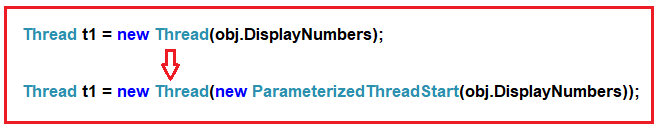##### Creating the ParameterizedThreadStart instance manually:

Let us see how to create the ParameterizedThreadStart delegate instance manually and passing that instance to the Thread class Constructor. So, modify the program as shown below.

```using System.Threading;
using System;
{
class Program
{
static void Main(string[] args)
{
Program obj = new Program();

t1.Start(5);

}

public void DisplayNumbers(object Max)
{
int Number = Convert.ToInt32(Max);
for (int i = 1; i <= Number; i++)
{
Console.WriteLine("Method1 :" + i);
}
}
}
}```

Now run the application and it should display the output as expected.

You need to use the ParameterizedThreadStart delegate if your method taking any values else you just need to use the ThreadStart delegate which does not take any parameter.

##### What are the Problems with the ParameterizedThreadStart delegate in C#?

As you can see, the parameter type of ParameterizedThreadStart delegate is object type. So, the parameter of the thread function is also going to be the object data type. And you cannot change the data type from object to any other type and if you try then it will give you a compile-time error. As the thread function operates on object data type now we can pass any type of values and it accepts. As a result, the function is not going to be type-safe as we can pass any type of values.

Let us try to pass a string value and see what happens as shown below.

```using System.Threading;
using System;
{
class Program
{
static void Main(string[] args)
{
Program obj = new Program();

t1.Start("Hi");
}

public void DisplayNumbers(object Max)
{
int Number = Convert.ToInt32(Max);
for (int i = 1; i <= Number; i++)
{
Console.WriteLine("Method1 :" + i);
}
}
}
}
```

Now you will not get any compile-time error, but once you run the application, then you will get runtime error as shown below.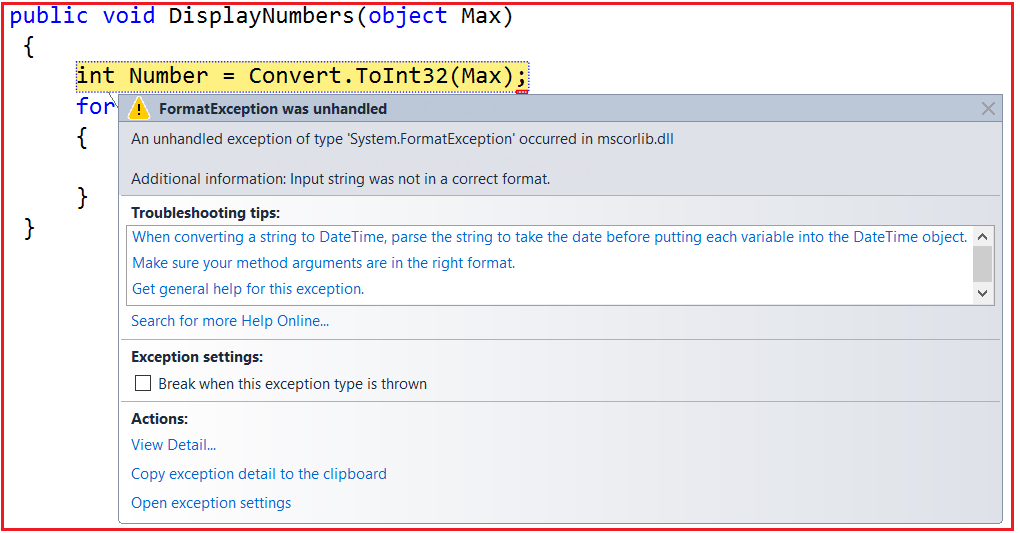That’s it for today, In the next article, I am going to discuss how to pass data to a thread function in a type-safe manner. Here, in this article, I try to explain the Constructors of Thread class in C# with examples. I hope you understood how to use the ThreadStart and ParameterizedThreadStart Delegates in Multithreading.